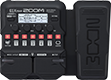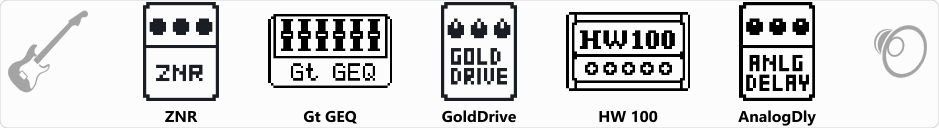# Plan A

Discussion in 'Zoom G1/G1X Four' started by Kev Vance, Aug 29, 2021.

1. Plan ADevice: Zoom G1X Four
Firmware: 2.00

Name on device: All Mine
Optimized for: Phones/Speaker

Effects chain:Based on my pedal board IRL

Effect: "ZNR" (Dynamics), active - "yes"
"Detect" = EFXIN
"Depth" = 59
"Threshold" = 49
"Decay" = 0

Effect: "Gt GEQ" (Filter), active - "yes"
"160" = -1.5
"400" = 0.0
"800" = 8.0
"3.2k" = 0.0
"6.4k" = 3.0
"12k" = 11.0
"VOL" = 80

Effect: "GoldDrive" (Overdrive / Distortion), active - "yes"
"Gain" = 76
"Bass" = 100
"Treble" = 42
"Volume" = 67

Effect: "HW 100" (Amp simulator), active - "yes"
"Input" = BRILL
"Bass" = 40
"Middle" = 80
"Treble" = 60
"Presence" = 60
"Gain" = 50
"Volume" = 60

Effect: "AnalogDly" (Delay), active - "yes"
"Time" = 3007
"F.B." = 17
"Mix" = 40
"Tail" = On

Patch Volume: 12